Inverse Laplace & Z-Transform of Rational Functions

# Inverse Laplace & Z-Transform of Rational Functions Notes | Study Signals and Systems - Electrical Engineering (EE)

## Document Description: Inverse Laplace & Z-Transform of Rational Functions for Electrical Engineering (EE) 2022 is part of Laplace and Z Transform for Signals and Systems preparation. The notes and questions for Inverse Laplace & Z-Transform of Rational Functions have been prepared according to the Electrical Engineering (EE) exam syllabus. Information about Inverse Laplace & Z-Transform of Rational Functions covers topics like and Inverse Laplace & Z-Transform of Rational Functions Example, for Electrical Engineering (EE) 2022 Exam. Find important definitions, questions, notes, meanings, examples, exercises and tests below for Inverse Laplace & Z-Transform of Rational Functions.

Introduction of Inverse Laplace & Z-Transform of Rational Functions in English is available as part of our Signals and Systems for Electrical Engineering (EE) & Inverse Laplace & Z-Transform of Rational Functions in Hindi for Signals and Systems course. Download more important topics related with Laplace and Z Transform, notes, lectures and mock test series for Electrical Engineering (EE) Exam by signing up for free. Electrical Engineering (EE): Inverse Laplace & Z-Transform of Rational Functions Notes | Study Signals and Systems - Electrical Engineering (EE)
 1 Crore+ students have signed up on EduRev. Have you?

Inverse Laplace transform :: Rational functions

Consider an arbitrary rational polynomial in Laplace Transform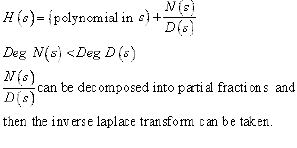Examples:

1) Let us consider the function in s: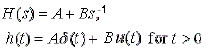2) Let us consider an LTI system with system function: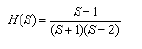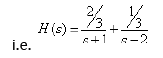As the ROC has not been specified. there are several different ROCs and correspondingly, several different system impulses. Possible ROCs for the system with poles at s = -1and s = 2 and a zero at s = 1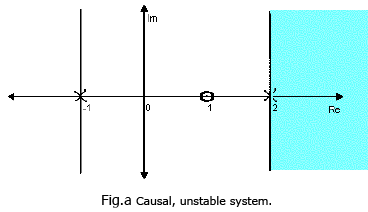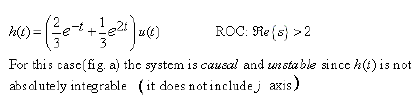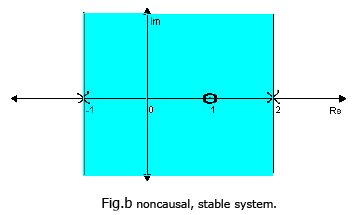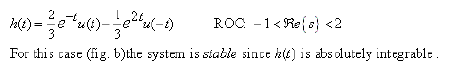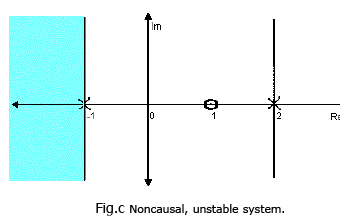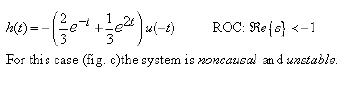Conclusions:

Properties of certain class of systems can be explained simply in terms of the locations of the poles. Particularly, consider a causal LTI system with a rational system function H(s). Since the system is causal, the ROC is to the right of the right most pole. Consequently, for this system to be stable (i.e. for the ROC to include the j-axis), the right most pole of H(s) must be to the left of the j-axis. i.e.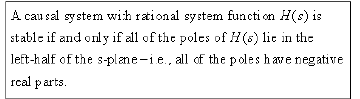Inverse Z - transform: Consider an arbitrary rational z-transform: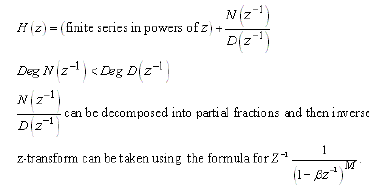Example 1:

Consider the z transform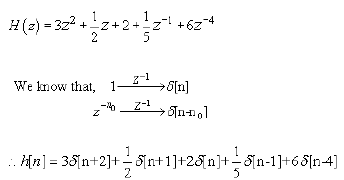Example :

Consider the z transform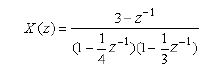There are two poles one at z=1/4 and at z=1/3. The partial fraction expansion, expressed in polynomials in 1/z, is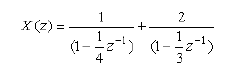Thus, x[n] is the sum of 2 terms, one with z - transform 1/[1-(1/4z)] and the other with z - transform 2/[1-(1/3z)]. Thus,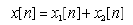As the ROC is not mentioned, we get different inverses for different possible ROCs. We do not discuss causality and stability as this may not be a system function. One possible inverse is worked out, the other two left as an exercise to the reader.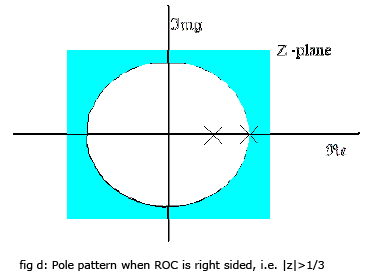We can identify by inspection ,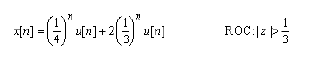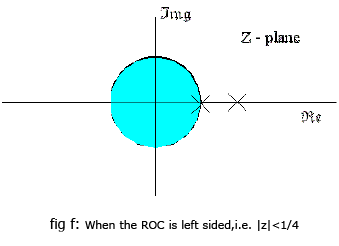Conclusion:

In this lecture you have learnt:

• if the system is causal then the ROC extends from the right most pole to infinity.
• A system is stable if the ROC includes the imaginary axis and therefore the right most pole of 'H(s)' must be to the left of the imaginary axis
• A causal system with a rational function 'H(s)' is stable if and only if all poles of H(s) lie in the left-half of the s-plane and must include the unit radius circle in the z-plane.

The document Inverse Laplace & Z-Transform of Rational Functions Notes | Study Signals and Systems - Electrical Engineering (EE) is a part of the Electrical Engineering (EE) Course Signals and Systems.
All you need of Electrical Engineering (EE) at this link: Electrical Engineering (EE)

## Signals and Systems

40 videos|41 docs|25 tests
 Use Code STAYHOME200 and get INR 200 additional OFF

## Signals and Systems

40 videos|41 docs|25 tests

Track your progress, build streaks, highlight & save important lessons and more!

,

,

,

,

,

,

,

,

,

,

,

,

,

,

,

,

,

,

,

,

,

;